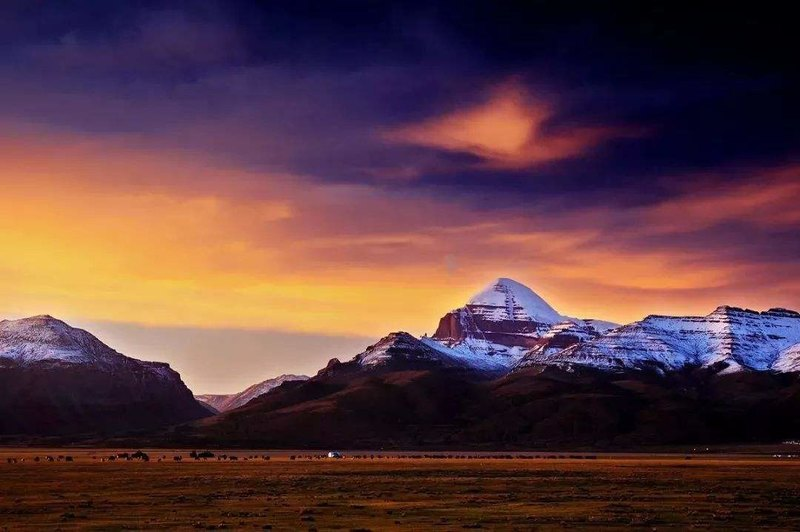# 中国最美十大名山

``1、南迦巴瓦(西藏)``

``2、珠穆朗玛(西藏)``

``3、冈仁波齐(西藏)````4、稻城三神山(四川)``

``5、贡嘎(四川)````6、峨眉山(四川)``

``7、梅里(云南)``

``8、黄山(安徽)````9、乔戈里(新疆)``

``10、泰山(山东)``

# 中国最美十大乡村

``1、丹巴藏寨(四川)``

``2、图瓦村(新疆)``

``3、哈尼村落(云南)````4、丽江大研镇(云南)``

``5、黎平肇兴侗寨(贵州)``

``6、婺源(江西)````7、漠河北极村(黑龙江)``

``8、白哈巴村(新疆)最佳季节：4月-11月``

``9、皖南古村落(安徽)``

``10、杏花村(安徽)``

# 中国最美十大森林

``1、天山雪岭云杉林(新疆)``

``2、轮台胡杨林(新疆)````3、波密岗乡灵芝云杉林(西藏)``

``4、西双版纳热带雨林(云南)````5、白马雪山高山杜鹃林(云南)``

``6、署南竹海(四川)``

``7、荔波喀斯特森林(贵州)````8、长白山红松阔叶混交林(吉林)``

``9、尖峰岭热带雨林(海南)``

``10、大兴安岭北部兴安落叶松林``